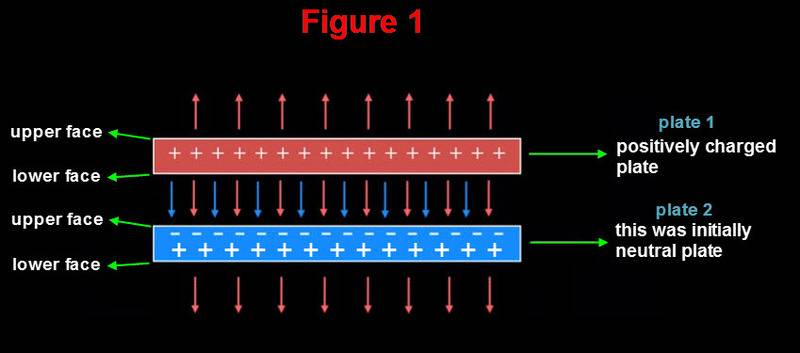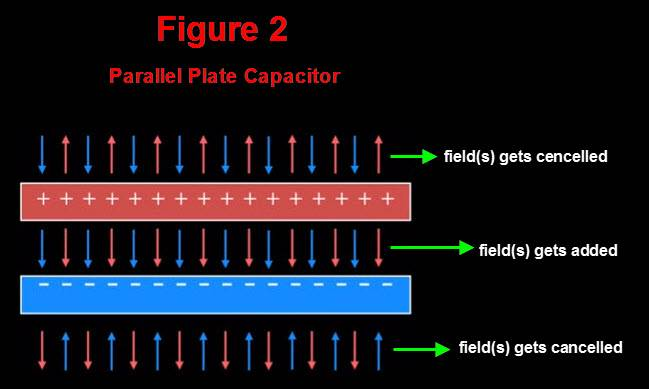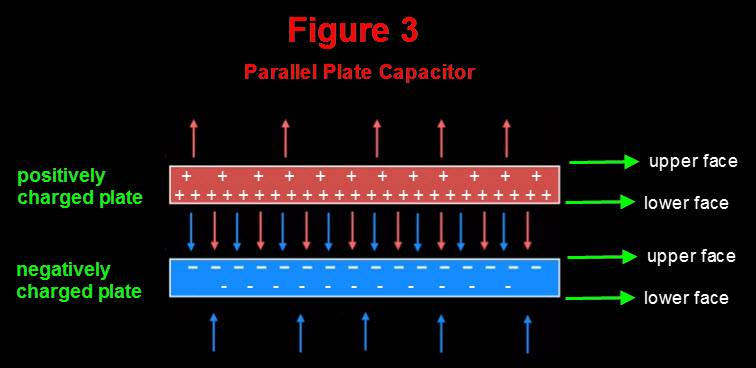# B Electric field outside a parallel plate capacitor

#### PainterGuy

Hi!

Could you please help me with the queries below? Thanks a lot.

Question 1:
My question is about the field outside the plates of parallel plate capacitor. It is said that the field(s) between positively and negatively charged plates gets added up but the field(s) outside the plates gets cancelled up. I don't understand how the external field(s) could get cancelled up.

Please have a look on this Figure #1 below.In the figure above plate 1 is positively charged and plate 2 was initially neutral. As plate 2 is exposed to the field of positively charged plate, the charge in it gets redistributed and one side of it becomes negatively charged and the other side becomes positively charged. We should note that number of 'positive' field lines emanating from the lower face of plate 2 is equal to the number of positive field lines entering upper face. Also, the both plates are electrically neutral between their upper and lower faces/boundaries. By the way, I believe that in a positively charged plate, plate 1, the charges would also redistribute in a way that the upper face would be less positively charged compared to the other one. I hope you agree with me up to this point.

Now please have a look on Figure #2 below. It shows how electric field of parallel plate capacitor is shown at many places though I don't agree that the field could get cancelled outside.In Figure #3 below I'm trying to show how in my opinion charges get redistributed once both positively and negatively charged plates are brought close together. I cannot understand how fields could get cancelled toward the upper face of positively charged plate and toward the lower face of negatively charged plate. Where am I going wrong?Question 2:
Electric for a parallel plate is given as shown below.
E=Q/(ε0A) where ε0 is vacuum permittivity and A is area of the plates
E=Vab/d where Vab is potential difference between the plates and 'd' is distance between them

I don't understand how reducing the distance between plates increases electric field. If the charge and area of plates don't change, 'd' shouldn't matter. It's not that reducing the 'd' would affect the charge, Q. Could you please guide me with this?

You could watch the video between 6:09 - 6:21. That section shows how 'd' affects electric field.

applet used in the video: https://phet.colorado.edu/en/simulation/capacitor-lab

Thank you!

#### Attachments

• 55.2 KB Views: 183
• 48.1 KB Views: 168
• 58.6 KB Views: 166

#### Lord Jestocost

Gold Member
2018 Award
Viewing at a charged capacitor from a certain distance, the capacitor as a whole turns out to be neutral. So, one experiences no electrical field owing to the capacitor.

Reducing the distance between the plates increases the electric field strength inside the capacitor when the external voltage source remains connected.

•PainterGuy

#### Nugatory

Mentor
I don't understand how reducing the distance between plates increases electric field. If the charge and area of plates don't change, 'd' shouldn't matter. It's not that reducing the 'd' would affect the charge, Q. Could you please guide me with this?
The electric field is the gradient of the voltage, analogous to the way that the slope of a ramp is the gradient of the height - if you have two ramps that are both one meter high, the shorter ramp will be steeper than the longer one.

With the capacitor, the voltage difference between the two plates doesn't change as you change the distance (it can't - both plates are still connected to the vltage source). But the field strength times the distance has to equal the voltage difference, so if you reduce the distance the field strength increases just as the ramp must get steeper if you make it shorter.

•PainterGuy

#### PainterGuy

Thank you, @Lord Jestocost, @Nugatory!

Viewing at a charged capacitor from a certain distance, the capacitor as a whole turns out to be neutral. So, one experiences no electrical field owing to the capacitor.
Yes, you are right that when a capacitor is looked at from a distance, it turns out to be neutral system. But my main question was more about when the capacitor is looked at from a closer distance. In other words, what figure between the two, Figure 2 and Figure 3, do you find correct? Many places I have been to online, use Figure 2. But I don't really understand how Figure 2 could be explained. In my opinion, Figure 3 correctly explains the electric field around the plates. By the way, while writing this post I checked a physics book by Young & Freedman and it uses a figure which looks more like Figure 3. Please have a look here: https://imageshack.com/a/img922/9967/VNJQP8.jpg

Reducing the distance between the plates increases the electric field strength inside the capacitor when the external voltage source remains connected.
With the capacitor, the voltage difference between the two plates doesn't change as you change the distance (it can't - both plates are still connected to the vltage source). But the field strength times the distance has to equal the voltage difference, so if you reduce the distance the field strength increases just as the ramp must get steeper if you make it shorter.
I understand what you are saying but I fail to understand how distance is important and why decreasing the distance would increase the electric field. In my opinion, electric field should only depend upon charge, Q, assuming area, A, is constant. Informally speaking, suppose there were 10 electric field lines when 'd' was 1 mm, then how making 'd' 0.5 mm would double the electric field lines?

Thanks for your help!

#### Nugatory

Mentor
In my opinion, electric field should only depend upon charge, Q, assuming area, A, is constant. Informally speaking, suppose there were 10 electric field lines when 'd' was 1 mm
"Field lines" are not an effective way of thinking about this stuff quantitatively. They provide a kinda-OK handwavy intuition in some problems, but the parallel plate capacitor is not one of them.

The magnitude of the electrical field at a point is a measure of how much the voltage changes over distance, just as the steepness of a ramp is a measure of how much the height changes over horizontal distance.

If the voltage difference between the plates is 10 volts and the plates are 10 mm apart, then the voltage must change by 1 volt per mm as you move from one plate to the other. If the plates are 1 mm apart, then the voltage must change by 10 volts per mm to get a full 10 volts of change between the two plates.

Another way of thinking about it: imagine a test particle with positive charge $Q$ at the bottom plate. How much work would you have to do on it to move it to the top plate? $Q\Delta{V}$, right? That's independent of the distance, as you're expecting. But that amount of work must also be equal to $W=Fd$ so the force you're acting against is dependent on the distance. But we also know that the electric field strength is proportional to the force by $F=QE$ so the field strength has to vary inversely with the distance.

The analogy with the ramp is that the total amount of energy needed to push an object to the top of the ramp depends only on the height ($W=mgh$). But a gentle ramp allows you to use a weaker force over a longer distance to end up with the same amount of $W=Fd$ work done.

•PainterGuy

#### Lord Jestocost

Gold Member
2018 Award
•PainterGuy

#### PainterGuy

Thank you, both of you!

### The Physics Forums Way

We Value Quality
• Topics based on mainstream science
• Proper English grammar and spelling
We Value Civility
• Positive and compassionate attitudes
• Patience while debating
We Value Productivity
• Disciplined to remain on-topic
• Recognition of own weaknesses
• Solo and co-op problem solving